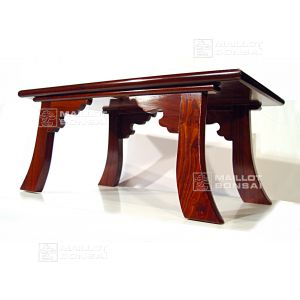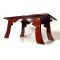##### The Japanese Bonsai specialist
Direct order Contact Help / Services Newsletter# Handmade bonsai exhibition table no.18

Bonsai dairef. : 4506

280,00

Available quantity : 1Order

###### Description

Length: 63 cm. Width: 34 cm. Height: 27.5 cm.

This grand-looking bonsai exhibition table is typical of the stands used in Japan to present conifers. The Asian style features curved legs and decorative corner brackets. Made by hand and assembled to a high standard.

#bonsai 5.6 #exhibition 4 #stands 3.6 #table 3.5 #handmade 2.8 #decorative 2 #assembled 1.9 #standard 1.8 #brackets 1.8 #conifers 1.8

Formule
(( ROUND((CHAR_LENGTH(b.article_nom)-CHAR_LENGTH(REPLACE(b.article_nom, 'exhibition', '')))/LENGTH('exhibition')) + ROUND((CHAR_LENGTH(b.article_description)-CHAR_LENGTH(REPLACE(b.article_description, 'exhibition', '')))/LENGTH('exhibition')) ) * 4) + (( ROUND((CHAR_LENGTH(b.article_nom)-CHAR_LENGTH(REPLACE(b.article_nom, 'bonsai', '')))/LENGTH('bonsai')) + ROUND((CHAR_LENGTH(b.article_description)-CHAR_LENGTH(REPLACE(b.article_description, 'bonsai', '')))/LENGTH('bonsai')) ) * 3.6) + (( ROUND((CHAR_LENGTH(b.article_nom)-CHAR_LENGTH(REPLACE(b.article_nom, 'table', '')))/LENGTH('table')) + ROUND((CHAR_LENGTH(b.article_description)-CHAR_LENGTH(REPLACE(b.article_description, 'table', '')))/LENGTH('table')) ) * 3.5) + (( ROUND((CHAR_LENGTH(b.article_nom)-CHAR_LENGTH(REPLACE(b.article_nom, 'handmade', '')))/LENGTH('handmade')) + ROUND((CHAR_LENGTH(b.article_description)-CHAR_LENGTH(REPLACE(b.article_description, 'handmade', '')))/LENGTH('handmade')) ) * 2.8) + (( ROUND((CHAR_LENGTH(b.article_nom)-CHAR_LENGTH(REPLACE(b.article_nom, 'decorative', '')))/LENGTH('decorative')) + ROUND((CHAR_LENGTH(b.article_description)-CHAR_LENGTH(REPLACE(b.article_description, 'decorative', '')))/LENGTH('decorative')) ) * 2) + (( ROUND((CHAR_LENGTH(b.article_nom)-CHAR_LENGTH(REPLACE(b.article_nom, 'assembled', '')))/LENGTH('assembled')) + ROUND((CHAR_LENGTH(b.article_description)-CHAR_LENGTH(REPLACE(b.article_description, 'assembled', '')))/LENGTH('assembled')) ) * 1.9) + (( ROUND((CHAR_LENGTH(b.article_nom)-CHAR_LENGTH(REPLACE(b.article_nom, 'standard', '')))/LENGTH('standard')) + ROUND((CHAR_LENGTH(b.article_description)-CHAR_LENGTH(REPLACE(b.article_description, 'standard', '')))/LENGTH('standard')) ) * 1.8) + (( ROUND((CHAR_LENGTH(b.article_nom)-CHAR_LENGTH(REPLACE(b.article_nom, 'brackets', '')))/LENGTH('brackets')) + ROUND((CHAR_LENGTH(b.article_description)-CHAR_LENGTH(REPLACE(b.article_description, 'brackets', '')))/LENGTH('brackets')) ) * 1.8) + (( ROUND((CHAR_LENGTH(b.article_nom)-CHAR_LENGTH(REPLACE(b.article_nom, 'conifers', '')))/LENGTH('conifers')) + ROUND((CHAR_LENGTH(b.article_description)-CHAR_LENGTH(REPLACE(b.article_description, 'conifers', '')))/LENGTH('conifers')) ) * 1.8) + (( ROUND((CHAR_LENGTH(b.article_nom)-CHAR_LENGTH(REPLACE(b.article_nom, 'features', '')))/LENGTH('features')) + ROUND((CHAR_LENGTH(b.article_description)-CHAR_LENGTH(REPLACE(b.article_description, 'features', '')))/LENGTH('features')) ) * 1.8)

## Secure payment## Delivery

Our logistic partners :